Type
Solution Manual
Book Title
Fundamentals of Investments: Valuation and Management 8th Edition
ISBN 13
978-1259720697

### 978-1259720697 Chapter 2 Solution Manual

January 2, 2020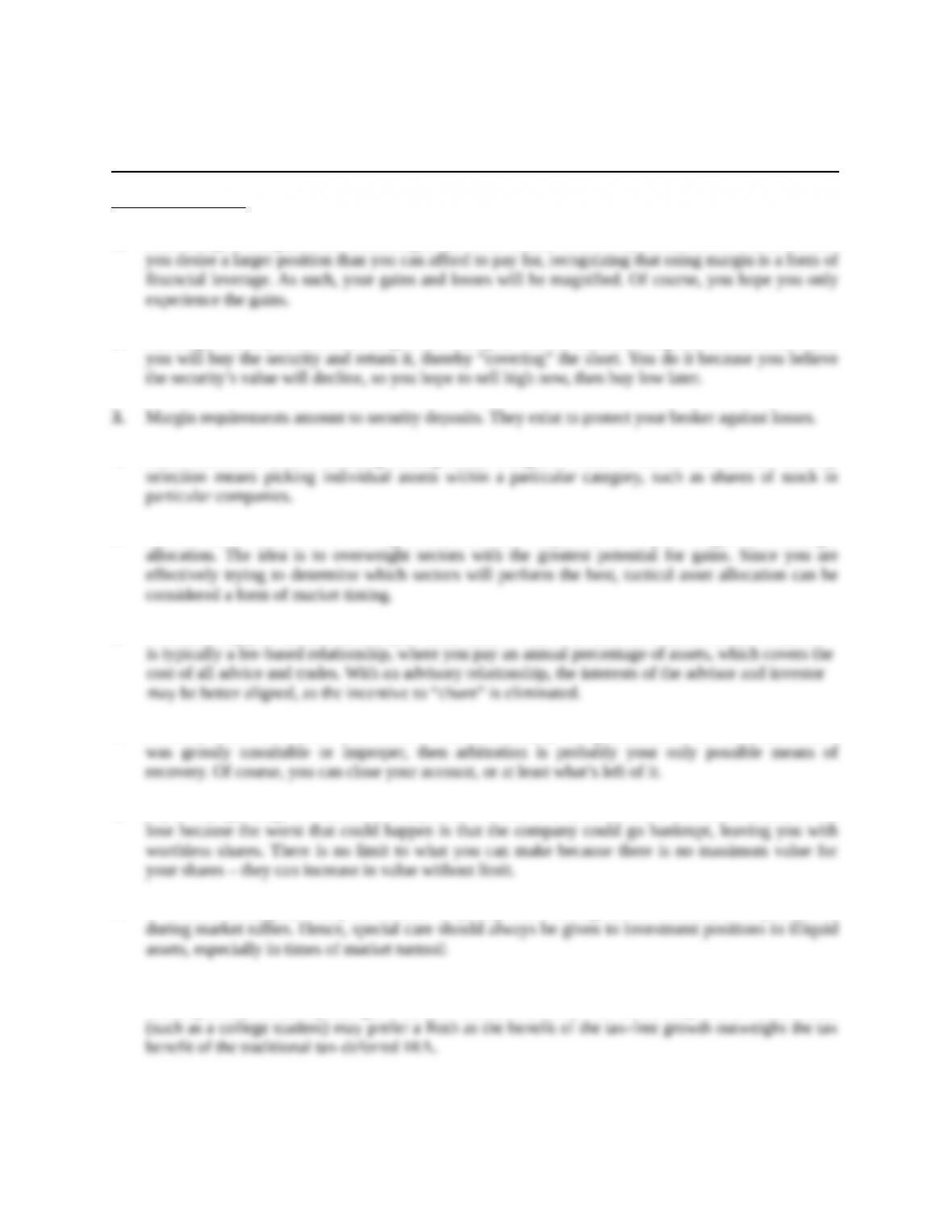Chapter 2
The Investment Process
Concept Questions
1. Purchasing on margin means borrowing some of the money used to buy securities. You do it because
2. Shorting a security means borrowing it and selling it, with the understanding that at some future date
4. Asset allocation means choosing among broad categories such as stocks and bonds. Security
5. Tactical asset allocation is making small, short-term adjustments to your longer-term strategic
7. Probably none. The advice you receive is unconditionally not guaranteed. If the recommendation
8. If you buy (go long) 500 shares at \$18, you have a total of \$9,000 invested. This is the most you can
9. If the asset is illiquid, it may be difficult to quickly sell it during market declines, or to purchase it
10. Traditional IRAs are tax-deferred, with withdrawals being taxed. Contributions to Roth IRAs are
taxed up-front, but all deposits grow tax free. Thus, an investor who is currently in a low tax bracket
Education.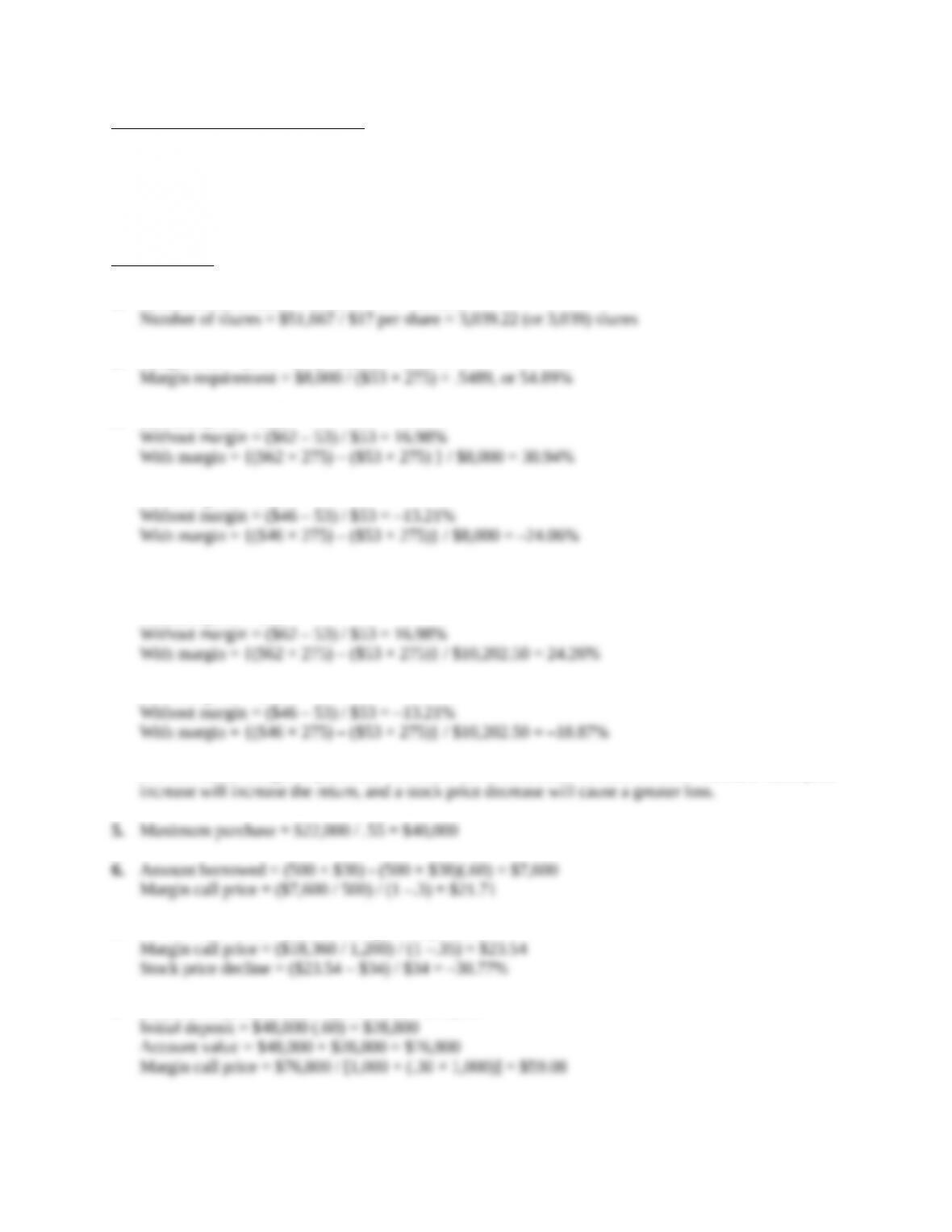Solutions to Questions and Problems
NOTE: All end of chapter problems were solved using a spreadsheet. Many problems require multiple
steps. Due to space and readability constraints, when these intermediate steps are included in this
solutions manual, rounding may appear to have occurred. However, the final answer for each problem is
found without rounding during any step in the problem.
Core questions
1. Maximum investment = \$31,000 / .60 = \$51,667
2. Margin loan = (\$53 × 275) – \$8,000 = \$6,575
3. Terminal price = \$62
Terminal price = \$46
4. Initial deposit = .70 × (\$53 × 275) = \$10,202.50
Terminal price = \$62
Terminal price = \$46
A lower initial margin requirement will make the returns more volatile. In other words, a stock price
7. Amount borrowed = (1,200 × \$34)(1 – .55) = \$18,360
8. Proceeds from short sale = 1,000 × \$48 = \$48,000
Education.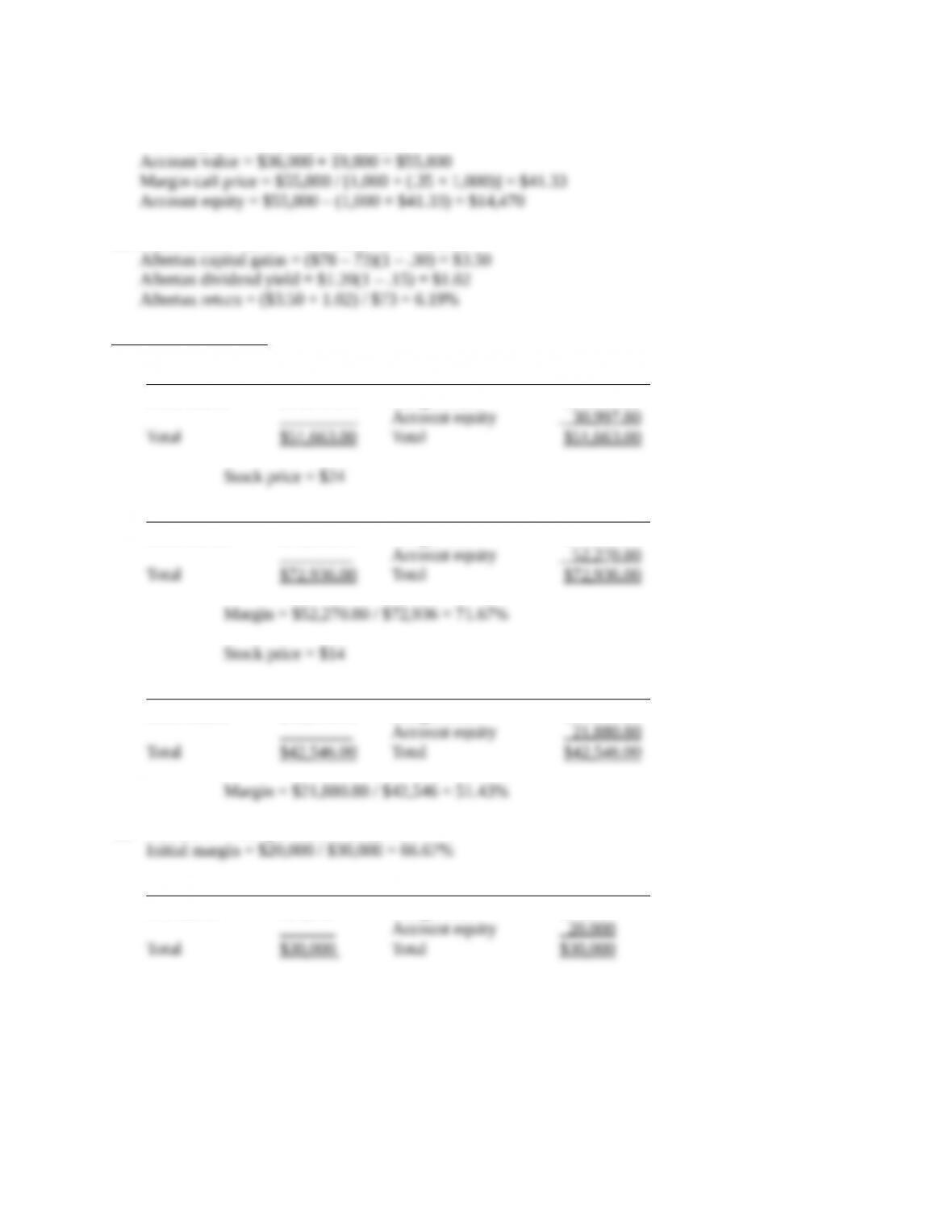9. Proceeds from short sale = 1,000(\$36) = \$36,000
Initial deposit = \$36,000(.55) = \$19,800
10. Pretax return = (\$78 – 73 + 1.20) / \$73 = 8.49%
Intermediate questions
11. Assets Liabilities and account equity
3039 shares \$51,663.00 Margin loan \$20,665.20
Assets Liabilities and account equity
3039 shares \$72,936.00 Margin loan \$20,665.20
Assets Liabilities and account equity
3039 shares \$42,546.00 Margin loan \$20,665.20
12. 500 shares × \$60 per share = \$30,000
Assets Liabilities and account equity
500 shares \$30,000 Margin loan \$10,000
Education.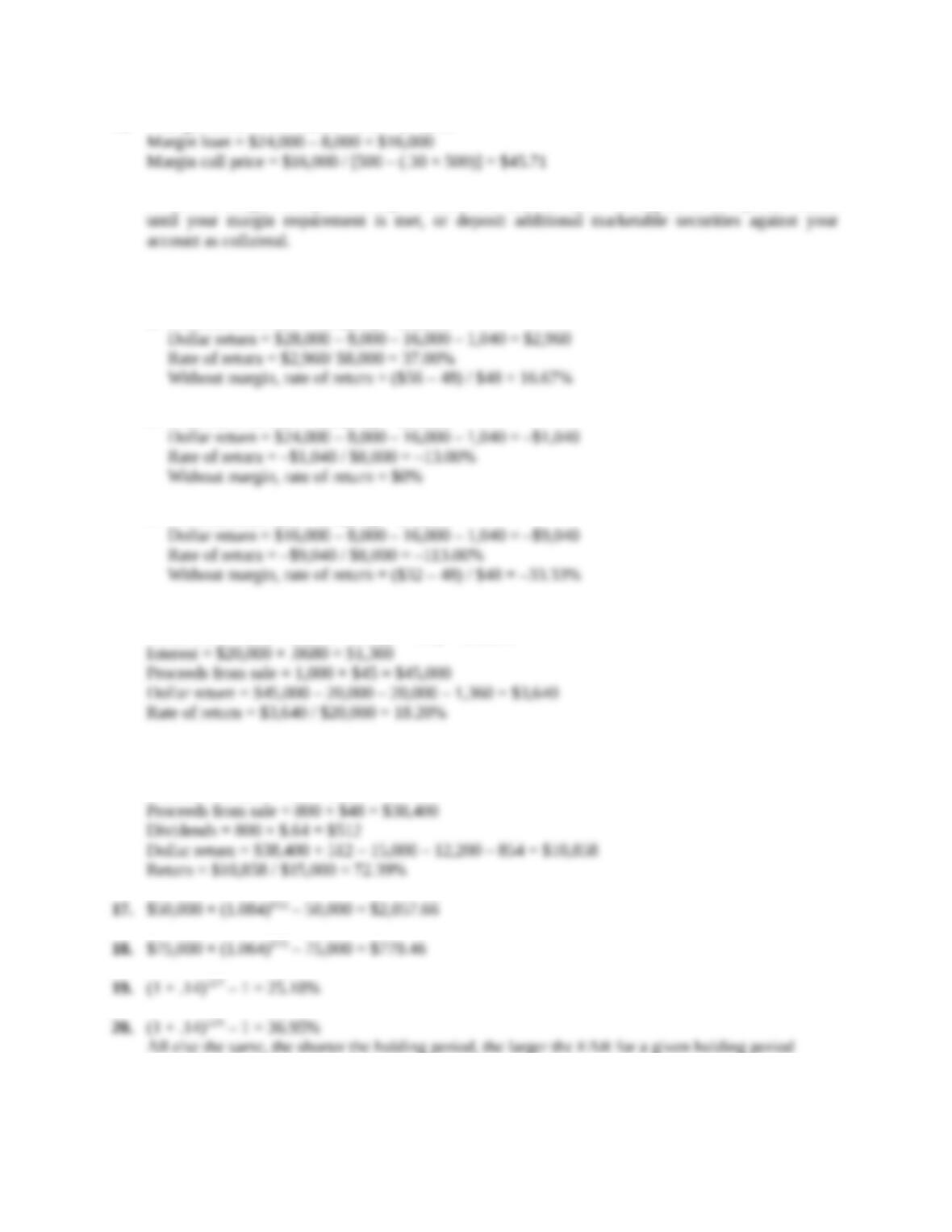13. Total purchase = 500 shares × \$48 = \$24,000
14. Interest on loan = \$16,000(1.065) – 16,000 = \$1,040
a. Proceeds from sale = 500(\$56) = \$28,000
b. Proceeds from sale = 500(\$48) = \$24,000
c. Proceeds from sale = 500(\$32) = \$16,000
15. Initial equity = (1,000 × \$40)(.50) = \$20,000
Amount borrowed = (1,000 × \$40)(1 – .50) = \$20,000
16. Total purchase = 800 × \$34 = \$27,200
Loan = \$27,200 – 15,000 = \$12,200
Interest = \$12,200 × .07 = \$854
All else the same, the shorter the holding period, the larger the EAR for a given holding period
return.
Education.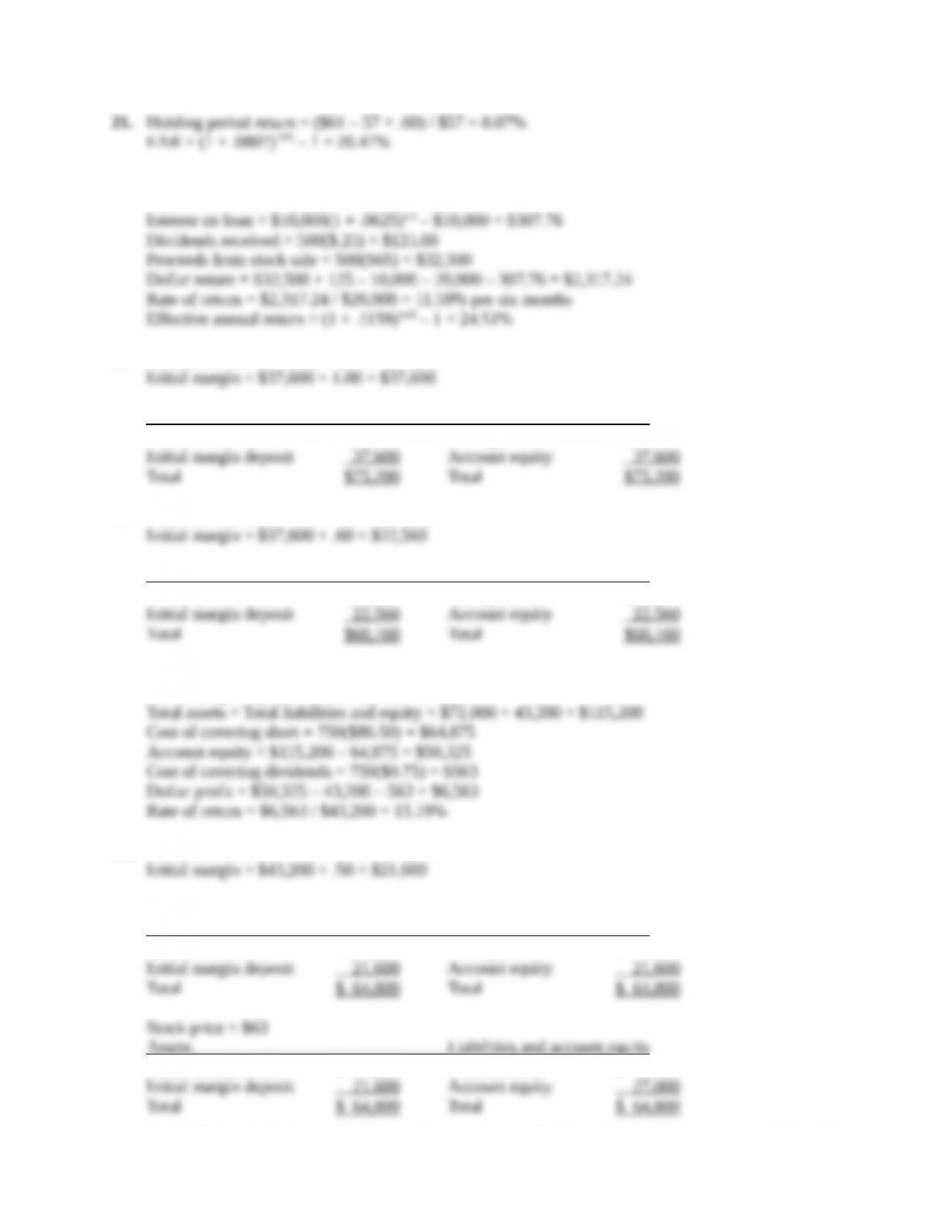22. Initial purchase = 500 × \$60 = \$30,000
Amount borrowed = \$30,000 – 20,000 = \$10,000
23. Proceeds from sale = 800 × \$47 = \$37,600
Assets Liabilities and account equity
Proceeds from sale \$37,600 Short position \$37,600
24. Proceeds from sale = 800 × \$47 = \$37,600
Assets Liabilities and account equity
Proceeds from sale \$37,600 Short position \$37,600
25. Proceeds from short sale = 750(\$96) = \$72,000
Initial margin deposit = \$72,000(.60) = \$43,200
26. Proceeds from sale = 600 × \$72 = \$43,200
Initial Balance Sheet
Assets Liabilities and account equity
Proceeds from sale \$ 43,200 Short position \$ 43,200
Assets Liabilities and account equity
Proceeds from sale \$ 43,200 Short position \$ 37,800
Education.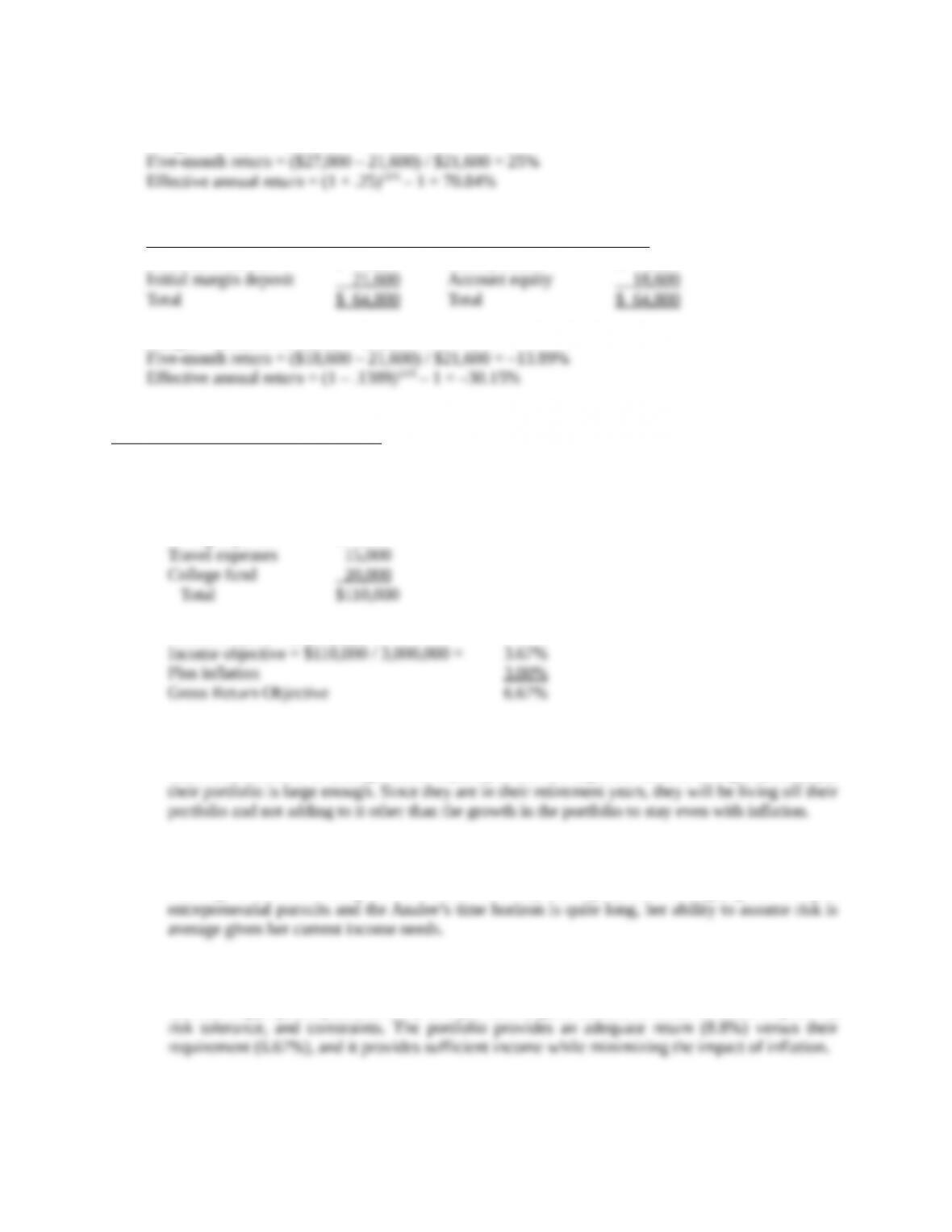Margin = \$27,000 / \$37,800 = 71.43%
Stock price = \$77
Assets Liabilities and account equity
Proceeds from sale \$ 43,200 Short position \$ 46,200
Margin = \$18,600 / \$46,200 = 40.26%
CFA Exam Review by Kaplan Schweser
1. a
The Analee’s pre-tax return objective is computed as follows:
Living expenses \$75,000
Portfolio Value = \$3,000,000
2. a
Their risk tolerance is average. Their liquidity needs are high due to their living expenses, yet
3. a
Although Barbara’s willingness to assume risk may be high (above average) given her past
4. a
The most appropriate portfolio is A, as it provides a good balance in terms of return objectives,